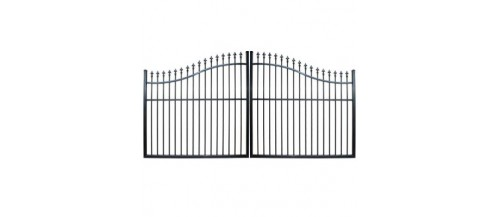Find us on Map and find Store Open Times# 1.8M high Arch Gates There are 16 products.

Showing 1 - 16 of 16 items
• ##### Arch Top Double Gate 3M (D52)

This gate is 3000mm wide and gate height at the end is 1520mm and height at middle is 1830mm.

\$ 800.00
In stock
• ##### Arch Top Double Gate 3.5M (D54)

This gate is 3500mm wide. Gate height at ends is 1520mm and height at middle is 1830mm.

\$ 900.00
In stock
• ##### Arch Top Double Gate 4.0M (D56)

This gate is 4000mm wide. Gate height at ends is 1520mm and height at middle is 1830mm.

\$ 1,100.00
In stock
• ##### Curved Top Double Gate 4.3M (D8)

This gate is 4267mm wide and gate height at the end is 1524mm and height at middle is 1828mm.

\$ 1,250.00
In stock
• ##### Curved Top Double Gate 4.9M (D9)

This gate is 4877mm wide and gate height at the end is 1524mm and height at middle is 1828mm.

\$ 1,450.00
In stock
• ##### Curved Top Double Gate 5.5M (D10)

This gate is 5486mm wide and gate height at the end is 1524mm and height at middle is 1828mm.

\$ 1,625.00 \$ 1,650.00
Reduced price!
In stock
• ##### Arch Top Double Gate 5.4M (D62)

This gate is 5400mm wide. Gate height at ends is 1520mm and height at middle is 1700mm.

\$ 1,900.00
In stock
• ##### Arch Top Double Gate 5.8M (D64)

This gate is 5800mm wide. Gate height at ends is 1520mm and height at middle is 1830mm.

\$ 2,100.00
In stock
• ##### Arch Top Double Gate 3M (D52C)

This gate is 3000mm wide and gate height at the end is 1520mm and height at middle is 1830mm.

\$ 1,150.00
In stock
• ##### Arch Top Double Gate 3.5M (D54C)

This gate is 3500mm wide and gate height at the end is 1520mm and height at middle is 1830mm.

\$ 1,375.00
In stock
• ##### Arch Top Double Gate 4.0M (D56C)

This gate is 4000mm wide and gate height at the end is 1520mm and height at middle is 1830mm.

\$ 1,600.00
In stock
• ##### Curved Top Double Gate 4.3M (D8C)

This gate is 4267M wide and gate height at the end is 1524M and height at middle is 1828M.

\$ 1,650.00
In stock
• ##### Curved Top Double Gate 4.9M (D9C)

This gate is 4877M wide and gate height at the end is 1524M and height at middle is 1828M.

\$ 1,850.00
In stock
• ##### Curved Top Double Gate 5.5M (D10C)

This gate is 5486M wide and gate height at the end is 1524M and height at middle is 1828M.

\$ 2,100.00
In stock
• ##### Arch Top Double Gate 5.4M (D62C)

This gate is 5400mm wide. Gate height at ends is 1520mm and height at middle is 1700mm.

\$ 2,500.00
In stock
• ##### Arch Top Double Gate 5.8M (D64C)

This gate is 5800mm wide. Gate height at ends is 1520mm and height at middle is 1830mm.

\$ 2,700.00
In stock
Showing 1 - 16 of 16 items